# Question:strange result using evalb() in debugger vs. outside debugger

## Question:strange result using evalb() in debugger vs. outside debugger

Maple 2021

I can't figure what is going on here. I have an expression, where when I run the program, I see inside the debugger that the result of evalb(simplify(tmp_1)) where tmp_1 is the expression, gives false

When I copy the same tmp_1 expression to new worksheet and do evalb(simplify(tmp_1)) it gives true.

I added print statements in the code to print tmp_1 and print result of simplify(tmp_1) and for some reason, it gives false when I run the program, but it gives true when I do the same thing in a work sheet, by copying tmp_1 and trying it there.

Never seen anything like this, as expression is all numbers. Here is the print out when I run the program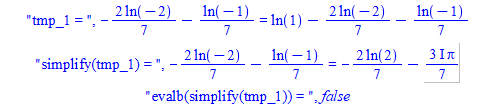Here is the same thing in worksheet

```restart;
tmp_1 :=-(2*ln(-2))/7 - ln(-1)/7 = ln(1) - (2*ln(-2))/7 - ln(-1)/7;
simplify(tmp_1);
evalb(simplify(tmp_1));
```

true

and if you think I made mistake somewhere, here is also screen shot from the debugger window itself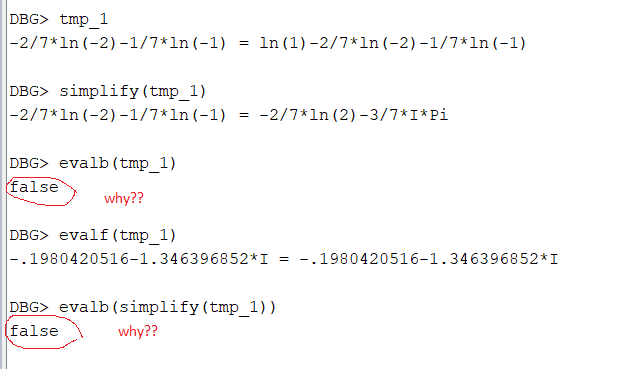again, same thing gives true in separate worksheet, copying same exact expression from above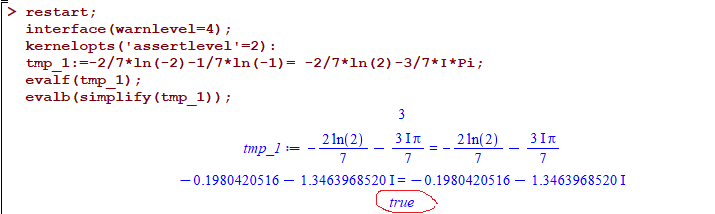There seem to be something loaded when I run my program that causes this difference, but I have no idea now what it is.  This has nothing to do with the debugger loaded btw, I am just using the debugger to show the details. When I run the program, without the debugger, I get false for the above, and I was trying to find why.

Any suggestions what to try and what to check for and what could cause this? Should it not give true when I run the program also? Could it be some Digits setting changed when the program run? I do not change any system defaults of any sort when running the program.

Maple 2020.1

edit june 12, 2021

Here is another manifestation of the same problem I just found. There is something seriously wrong for it to behave this way.

This is an expression called tmp_1 in a local variable in a proc inside a module. There are not even any local symbols in it. When I run the program, all the attempts to show it is true fail. Some give FAIL and some give false when the result should be true

When I simply copy the expression from the debugger window to an open worksheet, I get true

Here is the screen shot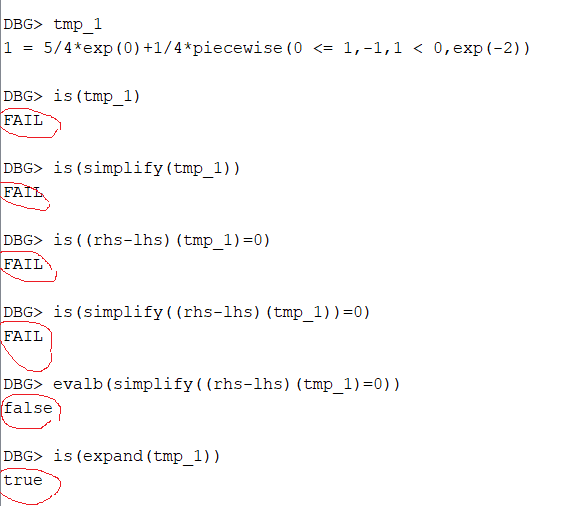Only when I did is(expand(tmp_1)) did it give true

But when I copy the expression to the worksheet, it gives true using all the other attempts:

```restart;
tmp_1:=1 = 5/4*exp(0)+1/4*piecewise(0 <= 1,-1,1 < 0,exp(-2)):
is(tmp_1);
is(simplify(tmp_1));
is((rhs-lhs)(tmp_1)=0);
is(simplify((rhs-lhs)(tmp_1)=0));
evalb(simplify((rhs-lhs)(tmp_1)=0));
is(expand(tmp_1))
```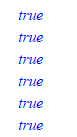I can't make a MWE so far, since these problems only show up with I run my large program. I think Maple internal memory get messed up or something else is loaded that causes these stranges problems.

For now, I added expand() to the things I should try. My current code now looks like

```if is(tmp_1) or is(simplify(tmp_1)) or is((rhs-lhs)(tmp_1)=0)
or is(simplify((rhs-lhs)(tmp_1)=0)) or evalb(simplify((rhs-lhs)(tmp_1)=0))
or is(expand(tmp_1)) then
....
```

Maple 2021.1﻿